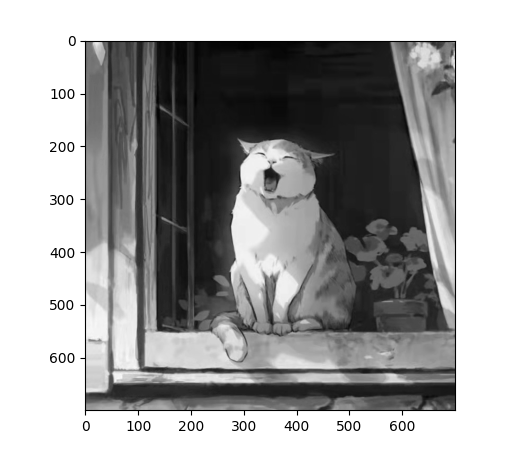• numpy数组转图像
2022-06-16 18:45:37

# 项目场景：pytorch生成图像

描述：np数组保存图像，使用matplotlib

下面展示一些 代码片

import matplotlib
matplotlib.image.imsave('name.png', array)
#我的array是32*32*3


# 问题描述

疑惑：如果用PIL的话，会报错attributeerror: module 'pil.image' has no attribute 'formarray'
不建议使用，也可能是我使用姿势不对

from PIL import Image
im = Image.fromarray(A)
im.save("your_file.jpeg")


疑惑：如果用PIL直接将tensor转为图像也有点问题'

# 参考链接

感谢：https://blog.csdn.net/zhuoyuezai/article/details/79635120pytorch python
更多相关内容
• 第一种方案 可以使用scipy.misc，代码如下： import scipy.misc misc.imsave('out.jpg', image_array) 上面的scipy版本会标准化所有图像，以便min(数据)变成...给定一个numpy数组”A”： from PIL import Image im =
• OpenCV中每张图像可以看成是 .array类型的二维或三维数组。8位的灰度图像是一个包含字节值的二维数组；8位的BGR图像是一个三维数组。例如：image[0,0] 或 image[0,0,0]第一个值代表像素的y坐标或行，0表示顶部；第二...

OpenCV中每张图像可以看成是 .array类型的二维或三维数组。8位的灰度图像是一个包含字节值的二维数组；8位的BGR图像是一个三维数组。

例如：

image[0,0] 或 image[0,0,0]

第一个值代表像素的y坐标或行，0表示顶部；

第二个值是像素的x坐标或列，0表示最左边；

第三个值(如果可用)表示颜色的通道。

1. 图像数组化

若一幅图的每个通道为8位，则可显式转换为标准的一维Python bytearray格式：byteArray = bytearray(image)

反之，bytearray含恰当顺序的字节，可以通过显式转换和重构，得到numpy.array形式图像：grayImage = np.array(grayByteArray).reshape(height,width)

bgrImage = np.array(bgrByteArray).reshape(height,width,3)

2. 随机数组转为图像

2.1 生成随机数组randomByteArray = bytearray(os.urandom(120000))

flatNumpyArray = np.array(randomByteArray)

2.2 转换为灰度图像

将随机数组转换为400*300灰色图像，保证元素个数相同grayImage = flatNumpyArray.reshape(300,400)

cv2.imwrite('RandomGray.png',grayImage)2.3 转换为彩色图像

将随机数组转换为400*100的彩色图像(注：彩色图像为3通道)bgrImage = flatNumpyArray.reshape(100,400,3)

cv2.imwrite('RandomColor.png',bgrImage)注意：本站所有文章除特别说明外，均为原创，转载请务必以超链接方式并注明作者出处。

展开全文• ## python 基于numpy数组的简单图像处理

千次阅读 多人点赞 2022-03-25 21:09:21
python 基于numpy数组的简单图像处理 如图，以该猫咪图片为例（忽略水印）。将该文件命名为cat.jpg，并对其展开以下操作。 使用PIL库进行灰度处理 from PIL import Image import numpy as np # 读取图像，并转化...

### 文章目录

python 基于numpy数组的简单图像处理

如图，以该猫咪图片为例（忽略水印）。将该文件命名为cat.jpg，并对其展开以下操作。# 使用PIL库进行灰度处理

from PIL import Image
import numpy as np

# 读取图像，并转化为数组
im = np.array(Image.open("cat.jpg"))

# 灰度处理公式
gray_narry = np.array([0.299, 0.587, 0.114])
x = np.dot(im, gray_narry)

# 数组转图片
gray_cat = Image.fromarray(x.astype('uint8'))
# 保存图片
gray_cat.save('gray_cat.jpg')
# 展示查看
gray_cat.show()


处理成功！# 将其旋转180度

所谓旋转操作，即 将三维数组中的数据，以每一行（三个）为单位 倒序排列。

from PIL import Image
import numpy as np

# 读取图像，并转化为数组
im = np.array(Image.open("cat.jpg"))
# 旋转
x = im[::-1]

# 数组转图片
cat2 = Image.fromarray(x.astype('uint8'))
# 保存图片
cat2.save('cat2.jpg')
# 展示查看
cat2.show()


程序执行结果：# 使用matplotlib库完成灰度处理

import numpy as np
import matplotlib.pyplot as plt
n1 = plt.imread("cat.jpg")  # 读取了图片，转化为数组，三维的
plt.imshow(n1)
n2 = np.array([0.299, 0.587, 0.114])
x = np.dot(n1, n2)
plt.imshow(x, cmap="gray")
plt.show()展开全文python 图像处理 灰度处理
• 我现在试图找出如何从base64数据中恢复numpy数组。这个问题和答案表明这是可能的：Reading numpy arrays outside of Python，但没有给出一个例子。以下面的代码为例，如果我知道数组的数据类型和形状，如何从base64...

我现在试图找出如何从base64数据中恢复numpy数组。这个问题和答案表明这是可能的：Reading numpy arrays outside of Python，但没有给出一个例子。

以下面的代码为例，如果我知道数组的数据类型和形状，如何从base64数据中获取Numpy数组？import base64

import numpy as np

t = np.arange(25, dtype=np.float64)

s = base64.b64encode(t)

r = base64.decodestring(s)

q = ?????

我希望python语句将q设置为dtype float64的numpy数组，因此结果是一个与t相同的数组。这就是编码和解码的数组的外观：>>> t = np.arange(25,dtype=np.float64)

>>> t

array([ 0., 1., 2., 3., 4., 5., 6., 7., 8., 9., 10.,

11., 12., 13., 14., 15., 16., 17., 18., 19., 20., 21.,

22., 23., 24.])

>>> s=base64.b64encode(t)

>>> s

>>> r = base64.decodestring(s)

>>> r

'\x00\x00\x00\x00\x00\x00\x00\x00\x00\x00\x00\x00\x00\x00\xf0?\x00\x00\x00\x00\x00\x00\x00@\x00\x00\x00\x00\x00\x00\x08@\x00\x00\x00\x00\x00\x00\x10@\x00\x00\x00\x00\x00\x00\x14@\x00\x00\x00\x00\x00\x00\x18@\x00\x00\x00\x00\x00\x00\x1c@\x00\x00\x00\x00\x00\x00 @\x00\x00\x00\x00\x00\x00"@\x00\x00\x00\x00\x00\x00\$@\x00\x00\x00\x00\x00\x00&@\x00\x00\x00\x00\x00\x00(@\x00\x00\x00\x00\x00\x00*@\x00\x00\x00\x00\x00\x00,@\x00\x00\x00\x00\x00\x00.@\x00\x00\x00\x00\x00\x000@\x00\x00\x00\x00\x00\x001@\x00\x00\x00\x00\x00\x002@\x00\x00\x00\x00\x00\x003@\x00\x00\x00\x00\x00\x004@\x00\x00\x00\x00\x00\x005@\x00\x00\x00\x00\x00\x006@\x00\x00\x00\x00\x00\x007@\x00\x00\x00\x00\x00\x008@'

>>> q = np.array( ????

我问这个问题的原因是因为我正在做一个项目，我想把很多Numpy数组存储在一个由django支持的应用程序的MySQL数据库中。

我想将数组作为base64写入数据库，而不是将数组转换为unicode字符串。

谢谢你的帮助。

展开全文• 在用python进行图像处理时，有时需要遍历numpy数组，下面是遍历数组的方法： [rows, cols] = num.shape for i in range(rows - 1): for j in range(cols-1): print(num[j, i]) 以上这篇Python遍历numpy数组的...
• OpenCV-Python Tutorials ...获取并修改像素值 读取一张图片，根据像素点的行和列的坐标获取它的像素值，对于BGR模式的图片，返回数组对应的值为BGR，对于灰度图则返回灰度值。 主要函数 img[row,co...opencv python 图像处理
• 用python显示一张图片方法如下：import matplotlib.pyplot as plt # plt 用于显示图片import matplotlib.image as mpimg # mpimg 用于读取图片import numpy as nplena = mpimg.imread('lena.png') # 读取和代码处于...
• Numpy数组保存为图像 https://blog.csdn.net/qq_37274615/article/details/79159468 有一个Numpy数组类型的矩阵，如何将它作为图像写入磁盘？任何格式的图像都行(PNG，JPEG，BMP ...)。 最佳解决办法 可以...
• Numpy数组的表达形式 每个维度是一个[]。 通道在前 通道在后
• 一、numpy array的存储和加载： load() 和 save() 函数是读写文件数组数据的两个主要函数，默认情况下，数组是以未压缩的原始二进制格式保存在扩展名为 .npy 的文件中。 savez() 函数用于将多个数组写入文件，默认...
• Numpy中使用ndim属性查看numpy数组的维度、使用shape属性查看numpy数组的形状机器学习 数据挖掘 人工智能
• Numpy 是 Python 中科学计算的核心库，NumPy 这...它提供了一个高性能的多维数组对象，以及大量的库函数和操作，可以帮助程序员轻松地进行数值计算，广泛应用于机器学习模型、图像处理和计算机图形学、数学任务等领域。
• opencv读取的图片类型为np.uint8 final_predictions[nb]是一个<class ‘numpy.ndarray’>类型 img = final_predictions[nb].astype(np.uint8)opencv python
• 图像处理来说，numpy数组是非常重要的。图像由像素值网格构成，numpy数组更像列表，更适合用于科学计算。 为什么需要numpy进行图像处理 当我们想将图像的亮度提升2倍，直接对列表进行乘法计算。 a = [1,2,3,4,5] b...python opencv
• 此python软件包提供了用于切片/修补和随后合并NumPy数组的功能。 对于各种繁重的图像处理任务（例如深度学习中的语义分割），特别是在图像不适合GPU内存的领域（例如，高光谱卫星图像，整个幻灯片图像，视频，断层...
• I have three (241, 241) numpy arrays which I would like to treat as the Red, Green and Blue components of an image.I have tried this:import numpy as npfrom PIL import Imagearr = np.zeros((len(x), len...
• import numpy as np from PIL import Image import matplotlib.pyplot as plt image=Image.open('cat.jpg') plt.imshow(image) plt.show() image=np.copy(image) # 这一句 print(image.shape) print(image)python
• Numpy支持常见的数组和矩阵操作。Numpy使用ndarray对象来处理多维数组，该对象是一个快速而灵活的大数据容器。 安装 windows: 升级 pip： python3 -m pip install -U pip 安装 Numpy库：（Ubuntu一样） pip install...python
• python 将 Numpy 数组保存为图像 - imagio： import numpy as np import imageio if __name__ == '__main__': np_array = np.zeros((512, 512, 3), dtype=np.uint8) np_array[200:300, 150:200, :] = 255 ...python imageio
• 0.给定一个numpy数组——image 1.使用opencv import cv2 cv2.imwrite("1.jpg", image) 2.使用PIL from PIL import Image im = Image.fromarray(image) im.save("1.jpg") 3.使用scipy.misc import scipy.misc scipy...opencv 计算机视觉 python
• # 注意下面QtGui.QImage的第四个参数，意思为图像每行有多少个字节，不设定时，图像有时会歪，所以一定要设定 im = QImage(im.data, im.shape, im.shape, im.shape*3, QImage.Format_RGB888) pix = ...python pyqt5
• 我的主程序检测到图像中的棋盘,并将其裁剪的图片传递给my_sliding_window方法.这应该使用Tensorflow来检测滑动窗口中的一块.从this教程我看到图片的内容如下：image_data = tf.gfile.FastGFile('picture.jpg', 'rb')......